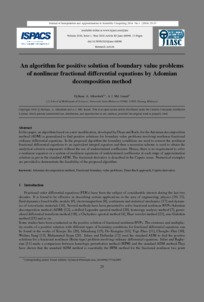# An algorithm for positive solution of boundary value problems of nonlinear fractional differential equations by Adomian decomposition method

A. I., Md. Ismail and Hytham. A., Alkresheh (2016) An algorithm for positive solution of boundary value problems of nonlinear fractional differential equations by Adomian decomposition method. Journal of Interpolation and Approximation in Scientific Computing, 2016 (1). pp. 25-37. ISSN 2194-3907Preview
PDF
Download (499kB) | Preview

## Abstract

In this paper, an algorithm based on a new modification, developed by Duan and Rach, for the Adomian decomposition method (ADM) is generalized to find positive solutions for boundary value problems involving nonlinear fractional ordinary differential equations. In the proposed algorithm the boundary conditions are used to convert the nonlinear fractional differential equations to an equivalent integral equation and then a recursion scheme is used to obtain the analytical solution components without the use of undetermined coefficients. Hence, there is no requirement to solve a nonlinear equation or a system of nonlinear equations of undetermined coefficients at each stage of approximation solution as per in the standard ADM. The fractional derivative is described in the Caputo sense. Numerical examples are provided to demonstrate the feasibility of the proposed algorithm.

Item Type: Article Q Science > QA Mathematics > QA1-939 Mathematics Pusat Pengajian Sains Matematik (School of Mathematical Sciences) > Article Mr Noorazilan Noordin 08 Jan 2018 07:41 08 Jan 2018 07:41 http://eprints.usm.my/id/eprint/38282

### Actions (login required)View Item